NEET  >  Test: Physics - 3

# Test: Physics - 3

Test Description

## 45 Questions MCQ Test NEET Mock Test Series | Test: Physics - 3

Test: Physics - 3 for NEET 2023 is part of NEET Mock Test Series preparation. The Test: Physics - 3 questions and answers have been prepared according to the NEET exam syllabus.The Test: Physics - 3 MCQs are made for NEET 2023 Exam. Find important definitions, questions, notes, meanings, examples, exercises, MCQs and online tests for Test: Physics - 3 below.
Solutions of Test: Physics - 3 questions in English are available as part of our NEET Mock Test Series for NEET & Test: Physics - 3 solutions in Hindi for NEET Mock Test Series course. Download more important topics, notes, lectures and mock test series for NEET Exam by signing up for free. Attempt Test: Physics - 3 | 45 questions in 45 minutes | Mock test for NEET preparation | Free important questions MCQ to study NEET Mock Test Series for NEET Exam | Download free PDF with solutions
 1 Crore+ students have signed up on EduRev. Have you?
Test: Physics - 3 - Question 1

### Given that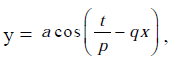where t represents time in second and x represents distance in metre. Which of the following statements is true ?

Test: Physics - 3 - Question 2

### The error in two readings of A are 0.01 and -0.03, then mean absolute error is ?

Detailed Solution for Test: Physics - 3 - Question 2

Solution :- Absolute mean error = (a1-a2)/2

[0.01-(-0.03)]/2

= 0.04/2

= 0.02.

Test: Physics - 3 - Question 3

### The angle between vectors (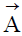X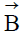) and (X) is

Test: Physics - 3 - Question 4
The sum, difference and cross product of two vectorsandare mutually perpendicular if
Test: Physics - 3 - Question 5

A boy is running over a circular track with uniform speed of 10 m/s. Find the average velocity for movement of boy from A to B. (in m/s)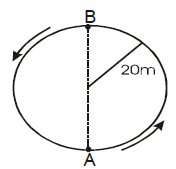Test: Physics - 3 - Question 6
A ball rolls from the top of a stair way with a horizontal velocity u m/s. If the steps are h metre high and b metre wide, the ball will hit edge of the nth step, if
Detailed Solution for Test: Physics - 3 - Question 6
Horizontal distance covered by ball =nb
Vertical distance covered by ball =nh
If the time taken to hit the edge of nth step is t, then
nb = ut
nh = 12gt^2
Eliminating t, we get,
nh = 1/2g (nb/u)^2
n = 2hu^2/gb^2
Test: Physics - 3 - Question 7
Which of the following is the altitude-time graph for a projectile thrown horizontally from the top of the tower?
Test: Physics - 3 - Question 8
Two blocks are connected over a massless pulley as shown in figure. The mass of block A is 10 kg and the coefficient of kinetic friction is 0.2. Block A slides down the incline at constant speed. The mass of block B in kg is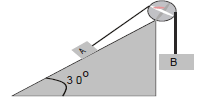Test: Physics - 3 - Question 9
Two masses m and M are attached with strings as shown. For the system to be in equilibrium we have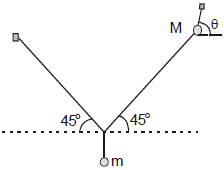Test: Physics - 3 - Question 10
A long spring is stretched by 2 cm. Its potential energy is U. If the spring is streched by 10 cm, its potential energy would be
Test: Physics - 3 - Question 11

A uniform flexible chain of mass m and length 2 l hangs in equilibrium over a smooth horizontal pin of negligible diameter. One end of the chain is given a small vertical displacement so that the chain slips over the pin.The speed of chain when it leaves pin is

Test: Physics - 3 - Question 12
A stone is tied with a string and is rotated in a circle horizontally. When the string suddenly breaks, the stone will move
Test: Physics - 3 - Question 13
A particle of mass m begins to slide down a fixed smooth sphere from the top. What is its tangential acceleration when it breaks off the sphere ?
Test: Physics - 3 - Question 14

A uniform circular disc of radius a is taken. A circular portion of radius b has been removed from it as shown in figure. If the centre of hole is at a distance c from the centre of the disc, the distance x2 of the centre of mass of the remaining part from the intial centre of mass O is given by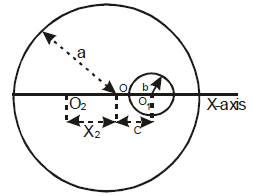Test: Physics - 3 - Question 15
A boy of mass m is standing on a block of mass M kept on a rough surface. When the boy walks from left to right on the block, the centre of mass (boy block) of system
Test: Physics - 3 - Question 16

The radius of gyration of a uniform rod of length L about on axis passing through its centre of mass is

Test: Physics - 3 - Question 17

Three thin rods each of length L and mass M are placed along x,y and z axis such that one end of each rod is at origin. The moment of inertia of this system about z-axis is

Test: Physics - 3 - Question 18
A ring and a disc having the same mass, roll without slipping with the same linear velocity. If the kinetic energy of the ring is 8J, that of the disc must be
Test: Physics - 3 - Question 19
In case of an orbiting satellite if the radius of orbit is decreased
Test: Physics - 3 - Question 20
For a satellite orbiting very close to earth’s surface total energy is
Test: Physics - 3 - Question 21

A point P (R √3,0,0) lies on the axis of a ring of mass M and radius R. The ring is located in y-z plane with its centre at origin O. A small particle of mass m starts from P and reaches O under gravitational attraction only. Its speed of O will be

Test: Physics - 3 - Question 22

A particle undergoing SHM has the equation : x = A sin (ω + φ ), where x represents the displacement of the particle. The kinetic energy oscillates with time period

Test: Physics - 3 - Question 23

The phase difference between the two simple harmonic oscillations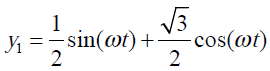y2 = sin (ωt) + cos (ωt)

Test: Physics - 3 - Question 24

Pressure versus temperature graph of an ideal gas of equal number of moles of different volumes are plotted as shown in figure. Choose the correct alternative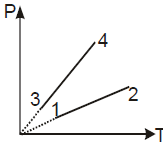Test: Physics - 3 - Question 25

A system undergoes a cyclic process in which it absorbs Q1 heat and gives out Q2 heat. The efficiency of the process is η and work done is W. Select the correct statement

Test: Physics - 3 - Question 26
A cyclic process for 1 mole of an ideal gas is shown in the V-T diagram. The work done in AB,BC and CA, respectively is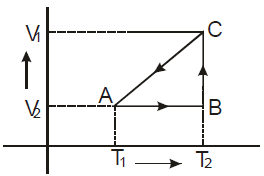Test: Physics - 3 - Question 27
Which of the following law states that “good absorbers of heat are good emitters” ?
Test: Physics - 3 - Question 28

A cylinder of radius R, made of a material of thermal conductivity k1, is surrounded by a cylindrical shell of inner radius R and outer radius 2R. The shell is made of a material of thermal conductivity k2. The two ends of the combined system are maintained at two different temperatures. There is no loss of heat across the cylindrical surface and the system is in steady state. The effective thermal conductivity of the system is

Test: Physics - 3 - Question 29
If you set up the seventh harmonic on a string fixed at both ends,how many nodes & antinodes are set up in it
Test: Physics - 3 - Question 30

A small source of sound moves on a circle as shown in figure and an observer in standing on O. Let n1, n2 and n3 be the frequencies heard when the source is at A,B and C respectively. Then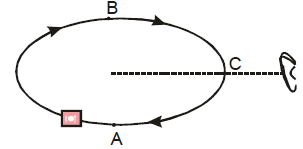Test: Physics - 3 - Question 31
For a certain orgain pipe, three successive resonance frequencies are observe at 425, 595 and 765 Hz. The speed of sound in air is 340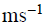. The pipe is a
Test: Physics - 3 - Question 32
Identify the figure, which correctly represented the given wave function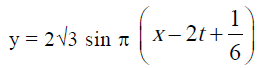Test: Physics - 3 - Question 33
The frequency of a note emitted by a source changes by 20% as it approaches stationary observer. As it recedes away from him the apparent frequency will be different from the actual frequency by (Velocity of sound in air is 340 m/s)
Test: Physics - 3 - Question 34
The linear density of a vibrating string is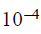kg/m. A transerve wave is propagating on the string, which is described by the equation y=0.02sin(x 30t) where x and y are in meters and the time t is in seconds. The tension in the string is
Test: Physics - 3 - Question 35
A point source of sound produces a sound level of 30dB at a distance of 8m from it. At what distance from the source the sound level will be 36 dB
Test: Physics - 3 - Question 36
A string of length 0.4 m and mass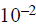kg is tightly clamped at its ends. The tension in the string is 1.6 N. Identical wave pulses are produced at one end at equal intervals of time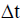. The minimum value of ?t which allows constructive interference between successive pulses is
Test: Physics - 3 - Question 37
Direction : Read the following question and choose
A. If both Assertion and Reason are correct and reason is the correct explanation of assertion
B. If both Assertion and Reason are true, but Reason is not correct explanation of the Assertion
C. If Assertion is true, but the Reason is false
D. If Assertion is false, but the Reason is true
E. If both assertion and reason are false

Assertion : To hear distinct beats, difference in frequencies of two sources should be less than 10
Reason : More the number of beats per sec more difficult to hear them
Test: Physics - 3 - Question 38
Direction : Read the following question and choose
A. If both Assertion and Reason are correct and reason is the correct explanation of assertion
B. If both Assertion and Reason are true, but Reason is not correct explanation of the Assertion
C. If Assertion is true, but the Reason is false
D. If Assertion is false, but the Reason is true
E. If both assertion and reason are false

Assertion : The bells are made of metals and not of wood
Reason : Wood offers high damping to the sound waves
Test: Physics - 3 - Question 39
Direction : Read the following question and choose
A. If both Assertion and Reason are correct and reason is the correct explanation of assertion
B. If both Assertion and Reason are true, but Reason is not correct explanation of the Assertion
C. If Assertion is true, but the Reason is false
D. If Assertion is false, but the Reason is true
E. If both assertion and reason are false

Assertion : For higher temperature, the peak emission wavelength of a black body shifts to lower wavelengths
Reason : Peak emission wavelength of a balck body is proportional to the fourth power of temperature
Test: Physics - 3 - Question 40

Direction : Read the following question and choose

A. If both Assertion and Reason are correct and reason is the correct explanation of assertion

B. If both Assertion and Reason are true, but Reason is not correct explanation of the Assertion

C. If Assertion is true, but the Reason is false

D. If Assertion is false, but the Reason is true

E. If both assertion and reason are false

Assertion : A room can be cooled by opening the door of a refrigator in a closed room

Reason : Heat flows from lower temperature (refrigerator) to higher temperature (room)

Test: Physics - 3 - Question 41
Direction : Read the following question and choose
A. If both Assertion and Reason are correct and reason is the correct explanation of assertion
B. If both Assertion and Reason are true, but Reason is not correct explanation of the Assertion
C. If Assertion is true, but the Reason is false
D. If Assertion is false, but the Reason is true
E. If both assertion and reason are false

Assertion : When a solid iron ball is heated, percentage increases is its volume is largest
Reason : Coefficient of superficial expansion is twice that of linear expansion where as coefficient of volume expansion is three time of linear expansion
Test: Physics - 3 - Question 42
Direction : Read the following question and choose
A. If both Assertion and Reason are correct and reason is the correct explanation of assertion
B. If both Assertion and Reason are true, but Reason is not correct explanation of the Assertion
C. If Assertion is true, but the Reason is false
D. If Assertion is false, but the Reason is true
E. If both assertion and reason are false

Assertion : The principle of superposition is not valid for gravitational force
Reason : Gravitational force is a conservative force
Test: Physics - 3 - Question 43
Direction : Read the following question and choose
A. If both Assertion and Reason are correct and reason is the correct explanation of assertion
B. If both Assertion and Reason are true, but Reason is not correct explanation of the Assertion
C. If Assertion is true, but the Reason is false
D. If Assertion is false, but the Reason is true
E. If both assertion and reason are false

Assertion : In an elastic collision of two bodies, the momentum and energy of each body is conserved
Reason : If two bodies stick to each other, after collision, the collision is said to be perfectly elastic
Test: Physics - 3 - Question 44
Direction : Read the following question and choose
A. If both Assertion and Reason are correct and reason is the correct explanation of assertion
B. If both Assertion and Reason are true, but Reason is not correct explanation of the Assertion
C. If Assertion is true, but the Reason is false
D. If Assertion is false, but the Reason is true
E. If both assertion and reason are false

Assertion : A cyclist always bends inwards while negotiating a curve
Reason : By bending he lower his centre of gravity
Test: Physics - 3 - Question 45
Direction : Read the following question and choose
A. If both Assertion and Reason are correct and reason is the correct explanation of assertion
B. If both Assertion and Reason are true, but Reason is not correct explanation of the Assertion
C. If Assertion is true, but the Reason is false
D. If Assertion is false, but the Reason is true
E. If both assertion and reason are false

Assertion : When the lift moves with uniform velocity the man in the lift will feel weightlessness
Reason : In downward accelerated motion of lift, apparent weight of a body decreases

## NEET Mock Test Series

1 videos|25 docs|146 tests
Information about Test: Physics - 3 Page
In this test you can find the Exam questions for Test: Physics - 3 solved & explained in the simplest way possible. Besides giving Questions and answers for Test: Physics - 3, EduRev gives you an ample number of Online tests for practice

## NEET Mock Test Series

1 videos|25 docs|146 tests

### How to Prepare for NEET

Read our guide to prepare for NEET which is created by Toppers & the best Teachers(Scan QR code)